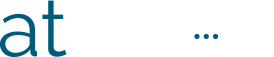#+ le mooc +

## Perform a simple calculation – Level 2

Palier Progress
0% Complete

You have just selected the relevant information to perform a calculation.

Another important step is to choose the mathematical operation you will use. Will you add, subtract, divide or multiply?

Before moving on to the exercises, we invite you to a short review that will help you understand the meaning of each operation in order to correctly choose the right one for each situation.

In the following quizzes, you will be asked to select the mathematical operation to be used in the proposed situation. Then you can perform the requested calculation.

Read the instructions carefully and imagine the situation to answer them correctly.

Quizzes